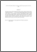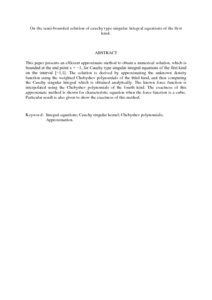# On the semi-bounded solution of cauchy type singular integral equations of the first kind.

## Citation

Mahiub, Mohammad Abdulkawi and Eshkuvatov, Zainidin K. and Nik Long, Nik Mohd Asri (2009) On the semi-bounded solution of cauchy type singular integral equations of the first kind. European Journal of Pure and Applied Mathematics, 2 (3). pp. 462-472. ISSN 1307-5543

## Abstract

This paper presents an efficient approximate method to obtain a numerical solution, which is bounded at the end point x = −1, for Cauchy type singular integral equations of the first kind on the interval [−1,1]. The solution is derived by approximating the unknown density function using the weighted Chebyshev polynomials of the third kind, and then computing the Cauchy singular integral which is obtained analytically. The known force function is interpolated using the Chebyshev polynomials of the fourth kind. The exactness of this approximate method is shown for characteristic equation when the force function is a cubic. Particular result is also given to show the exactness of this method.Preview
PDF (Abstract)
On the semi.pdfView Item